Home

# A Generalized Poisson Transform of an Lp -Function over the Shilov Boundary of the n-Dimensional Lie Ball

## Abstract

Let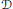$D$ be the$n$ -dimensional Lie ball and let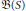$B\text{(S)}$ be the space of hyperfunctions on the Shilov boundary$S$ of$D$ . The aim of this paper is to give a necessary and sufficient condition on the generalized Poisson transform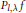${{P}_{l,\text{ }\!\!\lambda\!\!\text{ }}}f$ of an element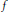$f$ in the space$B\text{(S)}$ for$f$ to be in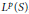${{L}^{p}}\left( S \right)$ ,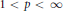$1\,<\,p\,<\,\infty$ . Namely, if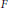$F$ is the Poisson transform of some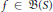$f\in \,B(S)$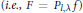$F\,=\,{{P}_{l,\lambda }}f$ ), then for any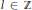$l\,\in \,Z$ ) and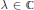$\lambda \,\in \,C$ such that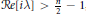$Re[\text{i}\lambda ] > \frac{n}{2}\,-\,1$ , we show that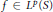$f\,\in \,{{L}^{p}}\text{(}S\text{)}$ if and only if$f$ satisfies the growth condition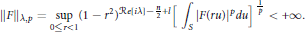$${{\left\| F \right\|}_{\lambda ,p}}=\underset{0\le r<1}{\mathop{\sup }}\,{{\left( 1\,-\,{{r}^{2}} \right)}^{\operatorname{Re}\left[ \text{i }\lambda \text{ } \right]-\frac{n}{2}+l}}{{\left[ \,\int_{s}{{{\left| F\left( ru \right) \right|}^{p}}du} \right]}^{\frac{1}{p}}}<\,+\infty$$

• # Send article to Kindle

Note you can select to send to either the @free.kindle.com or @kindle.com variations. ‘@free.kindle.com’ emails are free but can only be sent to your device when it is connected to wi-fi. ‘@kindle.com’ emails can be delivered even when you are not connected to wi-fi, but note that service fees apply.

Find out more about the Kindle Personal Document Service.

A Generalized Poisson Transform of an Lp -Function over the Shilov Boundary of the n-Dimensional Lie Ball
Available formats
×

# Send article to Dropbox

To send this article to your Dropbox account, please select one or more formats and confirm that you agree to abide by our usage policies. If this is the first time you use this feature, you will be asked to authorise Cambridge Core to connect with your <service> account. Find out more about sending content to Dropbox.

A Generalized Poisson Transform of an Lp -Function over the Shilov Boundary of the n-Dimensional Lie Ball
Available formats
×

# Send article to Google Drive

To send this article to your Google Drive account, please select one or more formats and confirm that you agree to abide by our usage policies. If this is the first time you use this feature, you will be asked to authorise Cambridge Core to connect with your <service> account. Find out more about sending content to Google Drive.

A Generalized Poisson Transform of an Lp -Function over the Shilov Boundary of the n-Dimensional Lie Ball
Available formats
×

## References

Hide All
MathJax
MathJax is a JavaScript display engine for mathematics. For more information see http://www.mathjax.org.

# A Generalized Poisson Transform of an Lp -Function over the Shilov Boundary of the n-Dimensional Lie Ball

## Metrics

### Full text viewsFull text views reflects the number of PDF downloads, PDFs sent to Google Drive, Dropbox and Kindle and HTML full text views.

Total number of HTML views: 0
Total number of PDF views: 0 *Loading metrics...

### Abstract viewsAbstract views reflect the number of visits to the article landing page.

Total abstract views: 0 *Loading metrics...

* Views captured on Cambridge Core between <date>. This data will be updated every 24 hours.

Usage data cannot currently be displayed